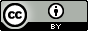Want to share your content on R-bloggers? click here if you have a blog, or here if you don't.

#### With `print()`

If you are an advanced R user, you probably know the lattice and the ggplot2 packages. They allow you to plot elegant graphics with less code (Hocking, 2009 and Sueur, 2010). If you know these two packages, you should hardly use `plot()` for your graphs.

If you read my article on FastRWeb, you may have wanted to try it with ggplot2 or lattice graphs. Your graphics have perhaps not been plotted. You did a Google search and you just learned you need the `plot()` function to properly display ggplot2 or lattice graphs with FastRWeb.

The code below shows how to reproduce the graph of `/var/FastRWeb/web.R/example1.png.R` with lattice and ggplot2.

```run <- function(...) {
"<meta charset='UTF-8'/></head><body><h1>Some plots…</h1>", sep = "")
X <- rnorm(100)
Y <- rnorm(100)

out("<h2>graphics</h2>")
p <- WebPlot(580, 580)
plot(x = X, y = Y, pch = 19)
out(p)

out("<h2>ggplot2</h2>")
require(ggplot2)
p <- WebPlot(580, 580)
print(qplot(x = X, y = Y, pch = 19))
out(p)

out("<h2>lattice</h2>")
require(lattice)
p <- WebPlot(580, 580)
print(xyplot(x = Y ~ X, pch = 19))
out(p)

out("</body></html>")
done()
}
```

With this script, you should see three graphics.

#### graphics#### ggplot2#### lattice##### References